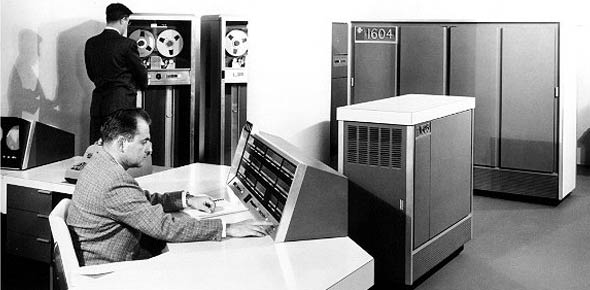# 3d1x2 5 Level CDC Vol. 1

69 Questions | Total Attempts: 1886Settings• 1.
What is an example of analog-to-digital and digital-to-analog techniques?
• A.

Amplitude modulation(AM) and freqeuncy modulation (FM)

• B.

Pulse code modulation (PCM) and frequency shift keying (FSK)

• C.

Phase modualtion (PM) and quadrature phase shift keying (QPSK)

• D.

Pulse code modulation (PCM) and continuously variable slope delta (CVSD) modulation

• 2.
What converts analog signal informaton into an analog carrier signal?
• A.

Delta modulation (DM)

• B.

Digital modulation

• C.

Analog modulation

• D.

• 3.
What converts analog signal informaton into a digital carrier signal?
• A.

Delta Modulation (DM)

• B.

Digital modulation

• C.

Analog modulation

• D.

• 4.
The information-carrying components that occur on either side of a carrier are called
• A.

Sidebands.

• B.

Sine waves.

• C.

Frequency translators.

• D.

Modulating fequencies.

• 5.
Amplitude modulation (AM) requires a bandwith that is
• A.

Twice that of the modulating signal.

• B.

Half that of the modulating signal.

• C.

Twice that of the carrier signal.

• D.

Half that of the carrire signal.

• 6.
The upper sideband in a single-sideband suppressed carrier (SSBSC) modulation is eliminated by
• A.

Filtering.

• B.

Detection.

• C.

Compression.

• D.

Companding.

• 7.
We can express the 6-to-1 improvement of the single-sideband suppressed carrier (SSBSC) mopdulation over conventional amplitude modulation (AM) as
• A.

2 decibels (dB) of improvement

• B.

4 decibels of improvement

• C.

6 decibels of improvement

• D.

8 decibels of improvement

• 8.
The only basic waveform in nature is a
• A.

Carrier wave.

• B.

Pure sine wave.

• C.

Resultant wave.

• D.

Composite wave.

• 9.
A modulated waveform that contains a carrier plus two sidebands for each modulation frrequency is a description of
• A.

A phase modulation (PM).

• B.

Pulse modulation.

• C.

A frequency modulation.

• D.

An amplitude modulation (AM).

• 10.
If 100 percent modulation is exceeded and there is distortion in the modulating signal, addititonal sidebands are generated. We indentify these sidebands as what?
• A.

Transmitted intelligence

• B.

Intensity of speech

• C.

Composite waves

• D.

Splatter

• 11.
The amount of deviation in a frquency modulation (FM) signal is directly proportinal to the modulating signal's
• A.

Phase.

• B.

Amplitude.

• C.

Frequency.

• D.

Apmlitude and frequency.

• 12.
The amount of phase shift in a phase modulation (PM) signal is directly proportional to the
• A.

Modulation index.

• B.

Rate of phase shift.

• C.

Frequency of the modulating signal.

• D.

Amplitude of the modulating signal.

• 13.
What form of modulation is insensitive to signal variations?
• A.

Delta

• B.

Frequency shift keying (FSK)

• C.

Pulse-amplitude modulation (PAM)

• D.

Binary-phaseshift keying or bi-phase shift keying (BPSK)

• 14.
In quadrature-phase shift keying (QPSK) the radio frequency (RF) bandwith for a quadriphase signal is what of that required by a bi-pahse signal, given the same data rate?
• A.

Four times

• B.

Double

• C.

Half

• D.

The same

• 15.
What is the main advantage of differential phase shift keying (DPSK)?
• A.

Carrier presence is required to detect change

• B.

It requires a less complex receiver than a basic phase shift keying (PSK) signal

• C.

It requires a more complex receiver than a basic PSK signal

• D.

The demodulator needds to detect changes in the absolute phase values

• 16.
Which of the following is not considered an analog modulation technique?
• A.

Pulse-amplitude modulation (PAM)

• B.

Pulse-duration modulation (PDM)

• C.

Pulse-positon modulation (PPM)

• D.

Pulse-code modulation (PCM)

• 17.
The form of pulse modulation in which the pulse is varied within a set range of positoins is known as
• A.

Pulse-amplitude modulation (PAM).

• B.

Pulse-duration modulation (PDM).

• C.

Pulse-positon modulation (PPM).

• D.

Pulse-code modulation (PCM).

• 18.
Quanitized analog modulation (QAM) is actually
• A.

Pulse-amplitude modulation (PAM).

• B.

Pulse-duration modulation (PDM).

• C.

Less tolerant of noise than frequency modulation (FM).

• D.

Analog-to-digital conversion

• 19.
How many bits per character of a 16-step pulse-code modulation (PCM) system are used to describe the quantized analog sample?
• A.

3

• B.

4

• C.

5

• D.

8

• 20.
The process of coding pulse code modulation (PCM) quantized pulses to make them a constant duration and amplitude is
• A.

Encoding.

• B.

Companding.

• C.

Uniform quantizing.

• D.

Nonuniform quantizing.

• 21.
In Time Division Multiplexing (TDM), the transmitting multiplexer will put  a bit or byte from each of the incoming lines into a specifically allocated what?
• A.

Channel

• B.

Trunk

• C.

Time slot

• D.

Port

• 22.
The two major types of frame structures are
• A.

Low speed and high speed

• B.

High speed and EBCDIC

• C.

CCITT and low speed

• D.

EBCDIC and CCITT

• 23.
The method of multiplexing the T1 multiplexer uses is
• A.

Frequency division.

• B.

Binary division.

• C.

Pulse division.

• D.

Time division.

• 24.
Wave-division multiplexing (WDM) creates teh virtual channels for multiplexing them together for transmission by using different
• A.

Wavelengths or lambas.

• B.

Wavelengths or gammas.

• C.

Frequencies or lambdas.

• D.

Frequencies or gammas.

• 25.
The most common wave-division multiplexing (WDM) system uses how many wavelengths?
• A.

1

• B.

2

• C.

3

• D.

4

Related TopicsBack to top